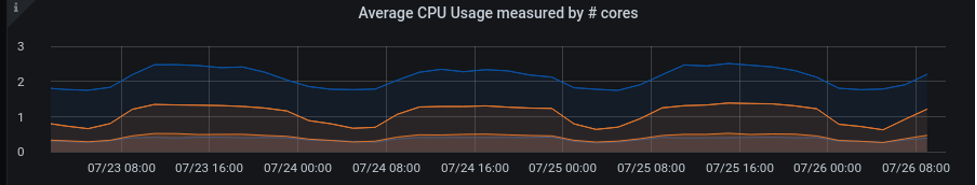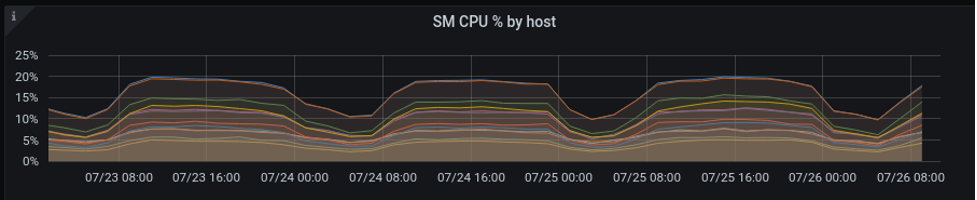# CPU Graphs

## Average CPU usage measured by the number of cores

This line graph plots the `total CPU time`, `User CPU time`, and `System CPU time` in units of cores for all Transaction Engines (TEs) and Storage Managers (SMs).Figure 1. Average CPU usage by measured number of cores

### Use Case

The `total CPU time`, `User time`, and `System time` for SMs and TEs are represented by different colors. Ideally, `User time` should be greater than `System time` and `total time` should be less than `total CPU cores`.

### Graph Creation Details

To create a graph using other tools, use the following details:

• TE Total: for ncores of `PercentCpuTime` filtered by TEs, average

• TE User: for ncores of `PercentUserTime` filtered by TEs, average

• TE System: for ncores of `PercentSystemTime` filtered by TEs, average

• SM Total: for ncores of `PercentCpuTime` filtered by SMs, average

• SM User: for ncores of `PercentUserTime` filtered by SMs, average

• SM System: for ncores of `PercentSystemTime` filtered by SMs, average

## Total CPU Usage measured by number of cores

This line graph plots the `total CPU usage`, `User CPU usage`, and `System CPU usage` each added together to indicate total CPU usage in number of cores.Figure 2. Total CPU usage measured by number of cores

### Use Case

`Total CPU time`, `User time`, and `System time` for SMs and TEs are represented by different colors. Ideally, `User time` should be greater than `System time` and `total time` should be less than `total CPU cores`.

### Graph Creation Details

To create a graph using other tools, use the following details:

• TE Total: (sum of ncores)/TE’s of `PercentCpuTime` filtered by all TE’s process, sum

• TE User: (sum of ncores)/TE’s of `PercentUserTime` filtered by all TE’s process, sum

• TE System: (sum of ncores)/TE’s of `PercentSystemTime` filtered by all TE’s process, sum

• SM Total: (sum of ncores)/SM’s of `PercentCpuTime` filtered by all SM’s process, sum

• SM User: (sum of ncores)/SM’s of `PercentUserTime` filtered by all SM’s process, sum

• SM System: (sum of ncores)/SM’s of `PercentSystemTime` filtered by all SM’s process, sum

## Average CPU percentage by Host for TEsFigure 3. Average CPU percentage by host for TEs

### Use Case

A single active TE should not be much higher than the others. Low CPU TEs in the earlier case are tie-breaker hosts (used for SM and TE majority decisions but not for running SQL). The `TE System CPU` should be much less than the `TE User CPU`.

### Graph Creation Details

To create a graph using other tools, use the following details:

• Total CPU: select norm of `PercentCpuTime` of TEs, average

• User CPU: select norm of `PercentUserTime` of TEs, average

• System CPU: select norm of `PercentSystemTime` of TEs, average

## Average CPU Percentage by Host for SMs

This line graph shows the average CPU usage, in percentage, by host for SMs.Figure 4. Average CPU percentage by host for SMs

### Use Case

A single SM should not be much higher than the others. `SM System CPU` should be much less than `SM User CPU`. Storage groups may change profiles. A Typical configuration has the same host specifications for all SMs.

### Graph Creation Details

To create a graph using other tools, use the following details:

• Total CPU: select norm of `PercentCpuTime` of SMs, average

• User CPU: select norm of `PercentUserTime` of SMs, average

• System CPU: select norm of `PercentSystemTime` of SMs, average

## Per Host CPU Percentage

Each host may have several SMs and TEs running. This line graph plots the sum of the processes on that host.Figure 5. Per Host CPU Percentage

### Use Case

`System CPU` should be much less than `User CPU`.

### Graph Creation Details

To create a graph using other tools, use the following details:

• Total CPU: sum of select norm from `PercentCpuTime`.

• User CPU: sum of select norm from `PercentUserTime`.

• System CPU: sum of select norm from `PercentSystemTime`.